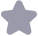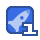### 最大熵阈值分割算法原始论文收藏 1

274 KAPUR SAHOO, AND WONG introduce a new algorithm (referred to as algorithm 3)and discuss its theoretical foundations. In Section 5, we give some examples of thresholding using the newly proposed method. Finally, in Section 6, we extend Algorithm 3 to"multithreshold ing 2. REFORMULATION AND ASSESSMENT OF ALGORITHM 1 Let fi,M2,.,f, be the observed gray-level frequencies and let ∑f=N, 1,2 where N is the total number of pixels in the picture and n is the number of gray-levels. The a posteriori entropy is defined by Hn=-PnP,-(1-P)n(1-P) (2) here P=∑p ∑ (3) If we maximize Hn, we get P=2 which gives an equal number of white and black pixels. To avoid this apparently trivial result we proceed as follows ∑plnp,≤∑pln[max(p1,P2…,P:) Py In(max( pi, P2,,Ps)I Hence ∑pnp;≥-Pln[max(1,p2…,P 1 us Implies H P≤ ln{max(p1,P2…,) where H.= P: n p THRESHOLDING USING ENTROPY 275 From (5), we get HIn P P,In P, max(Pi, p- Similarly (Hn-H3)n(1-P) P)n(1-P2)≤ tmax(P;+1,P4+2,…,P where ∑pnp ing(6)and(7)in(2), we H In(1-P B51如m(p,p2…,m+(-元 Pn Defining 五 ln(1-P2) Z/in[max(p1, P2,,p, I Hn in(max( Ps +1,P,+2., Pn)I and rewriting the above inequality, we obtain H ≤∫(s The function f(s) s)is called evaluation function [12 ]. According to Algorithm I the threshold value is the s which maximizes the evaluation function f(s). Due to an error in Eq( 18)of , Pun mistakenly obtained(8)as Hence H,f(s)gives the upper bound and not the lower bound of Hn, as claimed by Pun . Based on the inequality( 8)the following comments are in order (a) Let (s)=Hn/in, then from(8), we get φ(s)≤f(s) (10) The graph of p(s) has a fixed shape since it is related to Shannon s function throug cq. (2)whereas the graph of f(s) has no fixed shape. The dotted curves on Fig. 1 E represent three such arbitrary graphs of f(s). By Eq(4), the maximum of f(s)is 276 KAPUR, SAHOO, AND WONG fs 中(5) FiG.1. The shape of (s)and some possible shapes of /(5) always greater than or equal to that of p(s). However, there is no relationship between s,(the value of s at which the maximum of f(s)occurs)and s2(the value of s at which the maximum of p(s)occurs). \$2 always satisfies(4), but as is clear from Fig. 1, S, can be less than s,, equal to s2, or even greater than s, Based on his numerical calculation and using image data, Pun [ 13] found that s, is nearly the same as 5, and the closeness became pronounced as the smaller blocks of the picture are taken into account. This result is not altogether unexpected in view of the observation just made (b)The maximization of f(s)thus does not achieve a priori maximization of a posteriori entropy h (c)The reason why H should be maximized has not been explained (d) Since H is majorized by another function, therefore the algorithm does not lways use the statistical properties of the gray-level histogram (e)The function (s)can be majorized by sf(s),s f(s), or a(s)f(s),where a(s) is any positive function which is always greater than or equal to 1. But we cannot take the values of s at which these functions attain maxima as threshold value 3. ASSESSMENT OF ALGORITHM 2 We will first describe algorithm 2(for the convenience of the readers) and then critically examine its strength and weakness. Let the anisotropy coefficient a be defined by ∑pnp ∑p where m is the smallest integer for which P ifa≤0.5, 0.5 THRESHOLDING USING ENTROPY 277 In this algorithm, the lowest integer value m which divides the total frequencies into two or nearly two equal Parts is determined and then the ratio a of the entropy obtained for that partition is computed. If a 0.5 we choose s such that Ps=1-a If a <0.5, 1-a is greater than 0.5 and hence from( 12), we see that s >m. Again when a>0.5 the threshold value s is chosen such that P a. When a>0.5, from (12)s must be greater than m. When a =0.5, s is equal to m. Hence by this algorithm the threshold value is al ways greater than or equal to m. In general,nis algorithm produces less black than white pixels when"0"denotes the whiteness in the gray scale and thus introduces unnecessary bias This algorithm may give qualitatively sound results, but there is no guarantee that the results would always be satisfactory. In fact, a similar aniostropic heuristic algorithm could be obtained by considering the function ∑Pnp; g(s (13) ∑p|∑plp The threshold can be obtained by finding the value of s(sn)for which g(s) 15 nearer to unity. 4. THE NEW ALGORITHM In this section, we will propose a new algorithm based also on the entrop concept. Let p1 p2,,.,pn be the probability distrbution of gray-levels. Now we derive from this distribution two probability distributions, one defined for discrete values 1 to s and the other for values from s+ 1 to n. The two distributions are PI p2 (1 P, p P B P (15) 1-P The entropies associated with each distribution are as follows /I Pln p P ∑pnP1-PlnP3 In p+ H (16) KAPUR. SAHOO, AND WONG H(B)=-∑ P In P P ∑plnp1-(1-P)n(1-P,) H-H (1-P)+ Let us define the sum of H(A)and H(B)by y(s). Hence from(16)and(17),we obtain ↓(s)-lnP(1-P)+p+ We maximize y(s)to obtain the maximum information between the object and background distributions in the picture. The discrete value s which maximizes y(s) he threshold val If the distribution A and B are identical or similar, y (s)is maximum. If the distributions are uniform. then -3-+吗+二n1吗 In s(n-s and attains the maximum when s=,n. If the original gray level distribution (Pu P2,..., P)is symmetrical in the sense that PiPn 1,2,3, then for s =in(assume n even) H (1-P) H-H In P,t i.e., H(A)=H(B). And, in general, y(s)for symmetrical distribution will be at its maximum when s n 5. SOME EXPERIMENTAL RESULTS First we will discuss the choice of threshold value and then we will present the hreshold values of some real and artificially generated pictures. The graph of y(s) may have one of the following shapes(Figs. 2a-e): In Fig. 2a so is the obvious choice for threshold value. In Fig. 2b there is a choice between Sn and s2-In practice, we would like to maximize y(s), but we also want to have neither too many, nor too few, black pixels. If the nuber of black pixels corresponding to the threshold value 1 is sufficient, we choose s, otherwise we choose to have the threshold value larger than 5. Similarly in Figs. 2c-e we choose the threshold value THRESHOLDING USING ENTROPY 279 ψ(s ψ(s) yls) ys】▲ FIG.2. Some examples of the shape of y(s) by means of a suitable trade-off between maximizing y(s)and having a reasonable amount of black and white pixels for a good thresholding. Next we will describe our experimental results on four different pictures. These four pictures are (a)a cloud cover picture ( b)a building picture ,(c)a cameraman picture [123, and( d) pictures of a model We determine the threshold for hese pictures first without smoothing the gray-level requency data, and then with smoothing using the following formula  F(-2)+2F(i-1)+3F(i)+2F(i+1)+F(i+2 9 (22) It should be mentioned that for the following four experiments, the smoothing of the frequency data has no effect on the threshold value when Algonthm 3 is used (a) cloud cover picture. The data for this picture was taken from . Using Algorithm 3 the threshold value was found to bc 26 on a 0-63 gray scale. In their 280 KAPUR, SAHOO, AND WONG FIG 3. Original digitized building pictur FIG. 4. Gray level histogram of building picture. The arrow indicates the position of the threshold FIG. 5. Thresholded building picture. THRESHOLDING USING ENTROPY 281 FIG. 6. Original digitized picture of cameraman FIG. 7. Gray level histogram of picture of cameraman. The arrow indicates the position of the threshold val FIG 8. Threshold picture of cameraman

...展开详情

抢沙发
一个资源只可评论一次，评论内容不能少于5个字
l2472109912关注 私信 TA的资源
上传资源赚积分,得勋章
最新推荐最大熵阈值分割算法原始论文 36积分/C币 立即下载
1/14试读已结束，剩余9页未读...

36积分/C币 立即下载 ＞